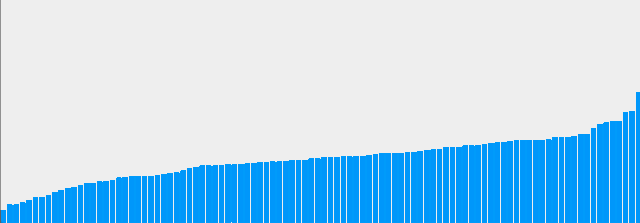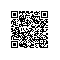# 一个有意思的分钱模拟问题

5
4
3
2
1// 初始化数据
money = new int;
for(int i = 0 ; i < money.length ; i ++)
money[i] = 100;for(int i = 0 ; i < money.length; i ++){
if(money[i] > 0){
int j = (int)(Math.random() * money.length);
money[i] -= 1;
money[j] += 1;
}
}Arrays.sort(money);
for(int i = 0 ; i < money.length; i ++){
if(money[i] > 0){
int j = (int)(Math.random() * money.length);
money[i] -= 1;
money[j] += 1;
}
}使用钉钉扫一扫加入圈子
+ 订阅